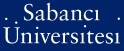# On the stability of 2^n-periodic binary sequences

Meidl, Wilfried (2005) On the stability of 2^n-periodic binary sequences. IEEE Transactions On Information Theory, 51 (3). pp. 1151-1155. ISSN 0018-9448PDF - Repository staff only - Requires a PDF viewer such as GSview, Xpdf or Adobe Acrobat Reader204Kb

Official URL: http://dx.doi.org/10.1109/TIT.2004.842709

## Abstract

The k-error linear complexity of a periodic binary sequence is defined to be the smallest linear complexity that can be obtained by changing k or fewer bits per period. This contribution focuses on the case of 2(n)-periodic binary sequences. For k = 1, 2, the exact formula for the expected k-error linear complexity of a sequence having maximal possible linear complexity 2(n), and the exact formula of the expected 1-error linear complexity of a random 2(n)-periodic binary sequence are provided. For k greater than or equal to 2, lower and upper bounds on the expected value of the k-error linear complexity of a random 2(n)-periodic binary sequence are established.

Item Type: Article cryptography; Chan-Games algorithm; (k-error) linear complexity; periodic sequences; stability of stream ciphers Q Science > QA Mathematics 677 Wilfried Meidl 22 Dec 2005 02:00 23 Sep 2009 11:09

Repository Staff Only: item control page